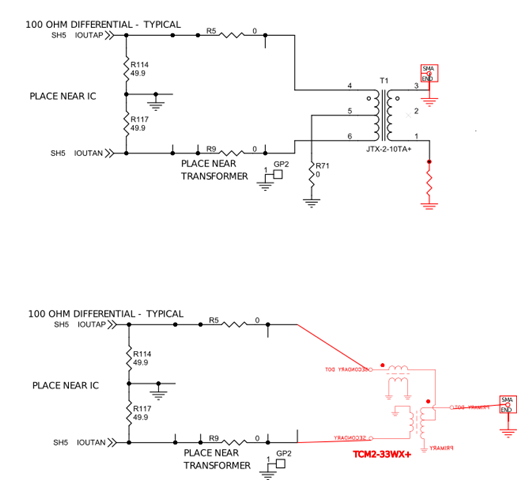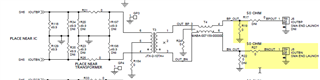If you have a related question, please click the "Ask a related question" button in the top right corner. The newly created question will be automatically linked to this question.

# DAC39J84: DAC39J84 output circuit

Part Number: DAC39J84

I want the DAC39J84 output converted to single ended SMA connector.

On the datasheet sec 7.3.28 and 7.3.29, it give two simple circuit for the DAC output single ended.

But on the evaluation board, it is using a 75 ohm balun (JTX-2-10TA+) followed by another Transmission line transformer (MABA-007159-000000).

1. Why use a 75 ohm balun in the evm board?

2. What are the benefit for the evaluation board configuration? Is the 2nd transformer mainly for balance the differential output using two SMAs? While I am only using single ended (1 SMA), I should not need that?

3. How would it compare with using the TCM-2-33WX (https://www.minicircuits.com/pdfs/TCM2-33WX+.pdf) reversely?

4. How about use a TCM4-19+ to convert the differential to single ended?

I am confused by all these different options and failed to analysis the pro and con for each of them.

Thanks

• Hi Gang,

The impedance of the balun or transformer is not so critical, you can always re-match the impedance as needed for the circuit to work appropriately.

The second balun/transformer is used to help balance the phase of the first balun/transformer in order to perserve the even order distortion at higher frequencies.

Choosing between the two balun/transformers you listed above really depends on the frequency band you are planning to use in your application. Either will work. But I would suggest to stay with 1:1 or 1:2 impedance ratios. 1:4 impedance ratios can be more difficult to use unless the BW is narrow and your application could use the extra voltage gain.

Regards,

Rob

• Hi Rob,

Thank you for answering this. I would like to follow up a little:

1. Regarding "The second balun/transformer is used to help balance the phase of the first balun/transformer in order to perserve the even order distortion at higher frequencies."

Is this only helpful if I use balanced output here ? If I simply use a single ended one SMA output, then that 2nd transformer doesn't do me any help, is that right?

2. For the DAC I am talking about (DAC39J84), the frequency output can be up to 1.4GHz, (2.8GSPS), I mainly plan to use it at lower side, +/- 1GHz or +/-500MHz. Can you elaborate some more on the  "1:4 impedance ratios can be more difficult to use unless the BW is narrow and your application could use the extra voltage gain."

3. Are these effects can be analyzed by some kind of simulation or purely from experience? If I do a simple simulation on transformer by spice, I will almost always get the perfect answer. Then how can I analyze this? Will load the DAC39J84 IBIS model to simulation show some difference ( I haven't done that)?

Thanks,

Gang

• Hi,

Just to chime in here: Minicircuits actually characterized the JTX-2-10TX balun for us in a 50ohm environment, and the performance holds. This is the reason that we had decided to go with the balun.

The IBIS mode contains actual measured parasitic R/L/C that may cause imabalance to the output. You may use such model to model the DAC output along with the balun. This is only at the output stage modeling the parasitic. The model does not have data for the actual DAC core performance reflected in the datasheet.

5141.JTX-2-10TA+_AP121094_RF_(5)_VM.PDF

• Hi,

Thank you for the information about the JTX-2-10TA+.

1. From the attached pdf, I can see they characterize to 1.6GHz and the phase unbalance is about 4 deg @(400M, >1.4G) . I am comparing with the TCM2-33WX+, which seems even a little better, I read about ~1.5@1.4G from the plot on datasheet. (https://www.minicircuits.com/pdfs/TCM2-33WX+.pdf)

And the TCM2-33WX+ is also cheaper and smaller.

2. I want single ended one SMA output, if I remove T2 in the evm and directly connect the T1 pin1 to ground and T1 pin 3 to SMA center pin. What kind of performance am I sacrificed?

3. If I used the two circuit as below, both start from the evm schematic DAC out side, the upper one still using the JTX2-10TX+, but remove the T2, the lower one use the TCM2-33WX+ instead, how will the circuit perform?4. Calculate the Vpp:

From the DAC39J84evm schematic, the RBIAS=1.91K. and the EXTIO is 0 by default. Coarse_dac=15 by default.

So the IOUTfs=(15+1)/16*0.9V/1.91Kohm*64=30mA

With these 2:1 transformer, if I still maintain IOUTfs= 30mA full scale output, the two 49.9 ohm resister are in parallel with the transformer resister, which is 50ohm x2

VOUTDIFF=30mA* ((49.9+49.9)//50*2) ohm=1.5V, voltage ratio of the 2:1 transformer is sqrt(2):1

So, I am going to get voltage Vpp=1.5/sqrt(2)=1.06 V in theory or about 4dBm on 50 ohm, is that the correct for the evm board or any output circuit using the 2:1 transformer?

But if VOUTDIFF=1.5, then the VOUTP or VOUTN will be +/- 0.75V to ground, while according to the section 6.1 in the datasheet, the absolute maximum ratings for the IOUTP and IOUTN are [-0.5,1.0V], the -0.75 side will damage the chip.

Thanks

•Hi

1. From the attached pdf, I can see they characterize to 1.6GHz and the phase unbalance is about 4 deg @(400M, >1.4G) . I am comparing with the TCM2-33WX+, which seems even a little better, I read about ~1.5@1.4G from the plot on datasheet. (https://www.minicircuits.com/pdfs/TCM2-33WX+.pdf)

The JTX-2-10x was selected as it can fit most of the 1st Nyquist zone coverage with the DAC sampling rate of 2.8GSPS. If you have a more narrow band applications and have found more suitable balun, please feel free to use it. You may contact Mini-circuits to see if they are willing to do some characterization of various performances over certain quantity of parts so you can get a feel for the variation.

2. I want single ended one SMA output, if I remove T2 in the evm and directly connect the T1 pin1 to ground and T1 pin 3 to SMA center pin. What kind of performance am I sacrificed?

potentially degradation in HD2 as the 2nd balun can shield the parasitic coupling of the transformer windings.

3. If I used the two circuit as below, both start from the evm schematic DAC out side, the upper one still using the JTX2-10TX+, but remove the T2, the lower one use the TCM2-33WX+ instead, how will the circuit perform?

circuit wise: no difference. You will need to test out the performance in your narrow band applications.

4. Calculate the Vpp:

From the DAC39J84evm schematic, the RBIAS=1.91K. and the EXTIO is 0 by default. Coarse_dac=15 by default.

So the IOUTfs=(15+1)/16*0.9V/1.91Kohm*64=30mA

With these 2:1 transformer, if I still maintain IOUTfs= 30mA full scale output, the two 49.9 ohm resister are in parallel with the transformer resister, which is 50ohm x2

VOUTDIFF=30mA* ((49.9+49.9)//50*2) ohm=1.5V, voltage ratio of the 2:1 transformer is sqrt(2):1

So, I am going to get voltage Vpp=1.5/sqrt(2)=1.06 V in theory or about 4dBm on 50 ohm, is that the correct for the evm board or any output circuit using the 2:1 transformer?

But if VOUTDIFF=1.5, then the VOUTP or VOUTN will be +/- 0.75V to ground, while according to the section 6.1 in the datasheet, the absolute maximum ratings for the IOUTP and IOUTN are [-0.5,1.0V], the -0.75 side will damage the chip.